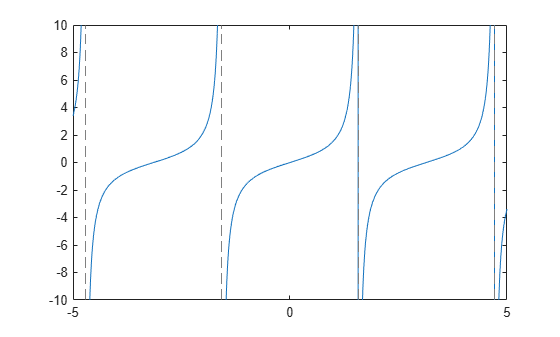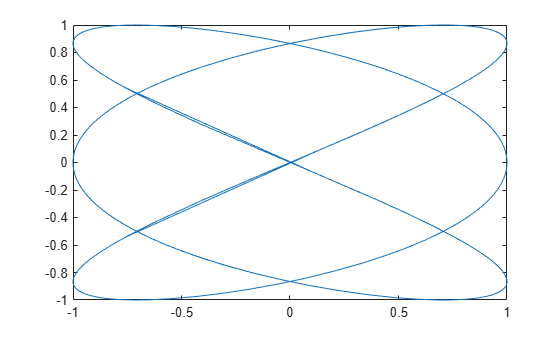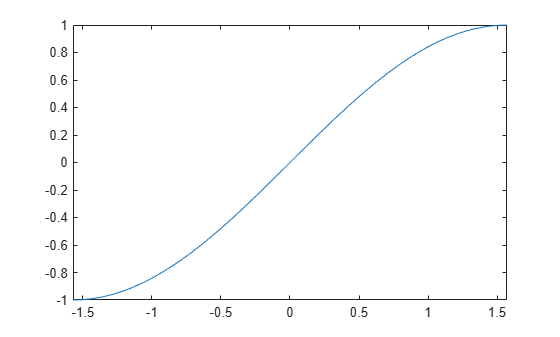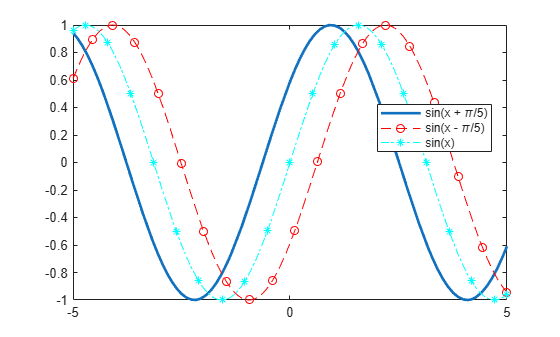Main Content

# fplot

Plot symbolic expression or function

## Syntax

fplot(f)
fplot(f,[xmin xmax])
fplot(xt,yt)
fplot(xt,yt,[tmin tmax])
fplot(___,LineSpec)
fplot(___,Name,Value)
fplot(ax,___)
fp = fplot(___)

## Description

example

fplot(f) plots symbolic input f over the default interval [-5 5].

example

fplot(f,[xmin xmax]) plots f over the interval [xmin xmax].

example

fplot(xt,yt) plots xt = x(t) and yt = y(t) over the default range of t, which is [–5 5].
fplot(xt,yt,[tmin tmax]) plots xt = x(t) and yt = y(t) over the specified range [tmin tmax].

example

fplot(___,LineSpec) uses LineSpec to set the line style, marker symbol, and line color.

example

fplot(___,Name,Value) specifies line properties using one or more Name,Value pair arguments. Use this option with any of the input argument combinations in the previous syntaxes. Name,Value pair settings apply to all the lines plotted. To set options for individual lines, use the objects returned by fplot.
fplot(ax,___) plots into the axes specified by ax instead of the current axes gca.

example

fp = fplot(___) returns a function line object or parameterized line object, depending on the type of plot. Use the object to query and modify properties of a specific line. For details, see FunctionLine Properties and ParameterizedFunctionLine Properties.

## Examples

### Plot Symbolic Expression

Plot tan(x) over the default range of [-5 5]. fplot shows poles by default. For details, see the ShowPoles argument in Name-Value Pair Arguments.

syms x fplot(tan(x))### Plot Symbolic Function

Plot the symbolic function $f\left(x\right)=\mathrm{cos}\left(x\right)$ over the default range [-5 5].

syms f(x) f(x) = cos(x); fplot(f)### Plot Parametric Curve

Plot the parametric curve $x=\mathrm{cos}\left(3t\right)$ and $y=\mathrm{sin}\left(2t\right)$.

syms t x = cos(3*t); y = sin(2*t); fplot(x,y)### Specify Plotting Interval

Plot $\mathrm{sin}\left(x\right)$ over $\left[-\pi /2,\pi /2\right]$ by specifying the plotting interval as the second input to fplot.

syms x fplot(sin(x),[-pi/2 pi/2])### Plot Multiple Lines on Same Figure

You can plot multiple lines either by passing the inputs as a vector or by using hold on to successively plot on the same figure. If you specify LineSpec and Name-Value arguments, they apply to all lines. To set options for individual plots, use the function handles returned by fplot.

Divide a figure into two subplots using subplot. On the first subplot, plot $\mathrm{sin}\left(x\right)$ and $\mathrm{cos}\left(x\right)$ using vector input. On the second subplot, plot $\mathrm{sin}\left(x\right)$ and $\mathrm{cos}\left(x\right)$ using hold on.

syms x subplot(2,1,1) fplot([sin(x) cos(x)]) title('Multiple Lines Using Vector Inputs') subplot(2,1,2) fplot(sin(x)) hold on fplot(cos(x)) title('Multiple Lines Using hold on Command') hold off### Change Line Properties and Display Markers

Plot three sine curves with a phase shift between each line. For the first line, use a linewidth of 2. For the second, specify a dashed red line style with circle markers. For the third, specify a cyan, dash-dot line style with asterisk markers. Display the legend.

syms x fplot(sin(x+pi/5),'Linewidth',2) hold on fplot(sin(x-pi/5),'--or') fplot(sin(x),'-.*c') legend('show','Location','best') hold off### Control Resolution of Plot

Control the resolution of a plot by using the MeshDensity option. Increasing MeshDensity can make smoother, more accurate plots, while decreasing it can increase plotting speed.

Divide a figure into two by using subplot. In the first subplot, plot a step function from x = 2.1 to x = 2.15. The plot's resolution is too low to detect the step function. Fix this issue by increasing MeshDensity to 39 in the second subplot. The plot now detects the step function and shows that by increasing MeshDensity you increased the plot's resolution.

syms x stepFn = rectangularPulse(2.1, 2.15, x); subplot(2,1,1) fplot(stepFn); title('Default MeshDensity = 23') subplot(2,1,2) fplot(stepFn,'MeshDensity',39); title('Increased MeshDensity = 39')### Modify Plot After Creation

Plot sin(x). Specify an output to make fplot return the plot object.

syms x h = fplot(sin(x))h = FunctionLine with properties: Function: [1x1 sym] Color: [0 0.4470 0.7410] LineStyle: '-' LineWidth: 0.5000 Show all properties 

Change the default blue line to a dashed red line by using dot notation to set properties. Similarly, add 'x' markers and set the marker color to blue.

h.LineStyle = '--'; h.Color = 'r'; h.Marker = 'x'; h.MarkerEdgeColor = 'b';### Add Title and Axis Labels and Format Ticks

For $x$ from $-2\pi$ to $2\pi$, plot $\mathrm{sin}\left(x\right)$. Add a title and axis labels. Create the x-axis ticks by spanning the x-axis limits at intervals of pi/2. Display these ticks by using the XTick property. Create x-axis labels by using arrayfun to apply texlabel to S. Display these labels by using the XTickLabel property.

To use LaTeX in plots, see latex.

syms x fplot(sin(x),[-2*pi 2*pi]) grid on title('sin(x) from -2\pi to 2\pi') xlabel('x') ylabel('y') ax = gca; S = sym(ax.XLim(1):pi/2:ax.XLim(2)); ax.XTick = double(S); ax.XTickLabel = arrayfun(@texlabel,S,'UniformOutput',false);### Re-evaluation on Zoom

When you zoom into a plot, fplot re-evaluates the plot automatically. This re-evaluation on zoom reveals hidden detail at smaller scales.

Plot x^3*sin(1/x) for -2 < x < 2 and -0.02 < y < 0.02. Zoom in on the plot using zoom and redraw the plot using drawnow. Because of re-evaluation on zoom, fplot reveals smaller-scale detail. Repeat the zoom 6 times to view smaller-scale details. To play the animation, click the image.

syms x fplot(x^3*sin(1/x)); axis([-2 2 -0.02 0.02]); for i=1:6 zoom(1.7) pause(0.5) end### Create Animations

Create animations by changing the displayed expression using the Function, XFunction, and YFunction properties and then by using drawnow to update the plot. To export to GIF, see imwrite.

By varying the variable i from 0.1 to 3, animate the parametric curve

$\begin{array}{l}x=it\mathrm{sin}\left(it\right)\\ y=it\mathrm{cos}\left(it\right).\end{array}$

To play the animation, click the image.

syms t fp = fplot(t, t); axis([-15 15 -15 15]) for i=0.1:0.05:3 fp.XFunction = i.*t.*sin(i*t); fp.YFunction = i.*t.*cos(i*t); drawnow end## Input Arguments

collapse all

Expression or function to plot, specified as a symbolic expression or function.

Plotting interval for x-coordinates, specified as a vector of two numbers. The default range is [-5 5]. However, if fplot detects a finite number of discontinuities in f, then fplot expands the range to show them.

Parametric input for x-coordinates, specified as a symbolic expression or function. fplot uses symvar to find the parameter.

Parametric input for y-axis, specified as a symbolic expression or function. fplot uses symvar to find the parameter.

Range of values of parameter t, specified as a vector of two numbers. The default range is [-5 5].

Axes object. If you do not specify an axes object, then fplot uses the current axes gca.

Line style, marker, and color, specified as a character vector or string containing symbols. The symbols can appear in any order. You do not need to specify all three characteristics (line style, marker, and color). For example, if you omit the line style and specify the marker, then the plot shows only the marker and no line.

Example: '--or' is a red dashed line with circle markers

Line StyleDescription
-Solid line
--Dashed line
:Dotted line
-.Dash-dot line
MarkerDescription
'o'Circle
'+'Plus sign
'*'Asterisk
'.'Point
'x'Cross
'_'Horizontal line
'|'Vertical line
's'Square
'd'Diamond
'^'Upward-pointing triangle
'v'Downward-pointing triangle
'>'Right-pointing triangle
'<'Left-pointing triangle
'p'Pentagram
'h'Hexagram
ColorDescription

y

yellow

m

magenta

c

cyan

r

red

g

green

b

blue

w

white

k

black

### Name-Value Pair Arguments

Specify optional comma-separated pairs of Name,Value arguments. Name is the argument name and Value is the corresponding value. Name must appear inside quotes. You can specify several name and value pair arguments in any order as Name1,Value1,...,NameN,ValueN.

Example: 'Marker','o','MarkerFaceColor','red'

The function line properties listed here are only a subset. For a complete list, see FunctionLine Properties.

Number of evaluation points, specified as a number. The default is 23. Because fplot uses adaptive evaluation, the actual number of evaluation points is greater.

Display asymptotes at poles, specified as 'on' or 'off', or as numeric or logical 1 (true) or 0 (false). A value of 'on' is equivalent to true, and 'off' is equivalent to false. Thus, you can use the value of this property as a logical value. The value is stored as an on/off logical value of type matlab.lang.OnOffSwitchState.

The asymptotes display as gray, dashed vertical lines. fplot displays asymptotes only with the fplot(f) syntax or variants, and not with the fplot(xt,yt) syntax.

Line color, specified as an RGB triplet, a hexadecimal color code, a color name, or a short name.

For a custom color, specify an RGB triplet or a hexadecimal color code.

• An RGB triplet is a three-element row vector whose elements specify the intensities of the red, green, and blue components of the color. The intensities must be in the range [0,1]; for example, [0.4 0.6 0.7].

• A hexadecimal color code is a character vector or a string scalar that starts with a hash symbol (#) followed by three or six hexadecimal digits, which can range from 0 to F. The values are not case sensitive. Thus, the color codes '#FF8800', '#ff8800', '#F80', and '#f80' are equivalent.

Alternatively, you can specify some common colors by name. This table lists the named color options, the equivalent RGB triplets, and hexadecimal color codes.

Color NameShort NameRGB TripletHexadecimal Color CodeAppearance
'red''r'[1 0 0]'#FF0000''green''g'[0 1 0]'#00FF00''blue''b'[0 0 1]'#0000FF''cyan' 'c'[0 1 1]'#00FFFF''magenta''m'[1 0 1]'#FF00FF''yellow''y'[1 1 0]'#FFFF00''black''k'[0 0 0]'#000000''white''w'[1 1 1]'#FFFFFF'Here are the RGB triplets and hexadecimal color codes for the default colors MATLAB® uses in many types of plots.

RGB TripletHexadecimal Color CodeAppearance
[0 0.4470 0.7410]'#0072BD'[0.8500 0.3250 0.0980]'#D95319'[0.9290 0.6940 0.1250]'#EDB120'[0.4940 0.1840 0.5560]'#7E2F8E'[0.4660 0.6740 0.1880]'#77AC30'[0.3010 0.7450 0.9330]'#4DBEEE'[0.6350 0.0780 0.1840]'#A2142F'Example: 'blue'

Example: [0 0 1]

Example: '#0000FF'

Line style, specified as one of the options listed in this table.

Line StyleDescriptionResulting Line
'-'Solid line'--'Dashed line':'Dotted line'-.'Dash-dotted line'none'No lineNo line

Line width, specified as a positive value in points, where 1 point = 1/72 of an inch. If the line has markers, then the line width also affects the marker edges.

The line width cannot be thinner than the width of a pixel. If you set the line width to a value that is less than the width of a pixel on your system, the line displays as one pixel wide.

Marker symbol, specified as one of the values listed in this table. By default, the object does not display markers. Specifying a marker symbol adds markers at each data point or vertex.

ValueDescription
'o'Circle
'+'Plus sign
'*'Asterisk
'.'Point
'x'Cross
'_'Horizontal line
'|'Vertical line
'square' or 's'Square
'diamond' or 'd'Diamond
'^'Upward-pointing triangle
'v'Downward-pointing triangle
'>'Right-pointing triangle
'<'Left-pointing triangle
'pentagram' or 'p'Five-pointed star (pentagram)
'hexagram' or 'h'Six-pointed star (hexagram)
'none'No markers

Marker outline color, specified as 'auto', an RGB triplet, a hexadecimal color code, a color name, or a short name. The default value of 'auto' uses the same color as the Color property.

For a custom color, specify an RGB triplet or a hexadecimal color code.

• An RGB triplet is a three-element row vector whose elements specify the intensities of the red, green, and blue components of the color. The intensities must be in the range [0,1]; for example, [0.4 0.6 0.7].

• A hexadecimal color code is a character vector or a string scalar that starts with a hash symbol (#) followed by three or six hexadecimal digits, which can range from 0 to F. The values are not case sensitive. Thus, the color codes '#FF8800', '#ff8800', '#F80', and '#f80' are equivalent.

Alternatively, you can specify some common colors by name. This table lists the named color options, the equivalent RGB triplets, and hexadecimal color codes.

Color NameShort NameRGB TripletHexadecimal Color CodeAppearance
'red''r'[1 0 0]'#FF0000''green''g'[0 1 0]'#00FF00''blue''b'[0 0 1]'#0000FF''cyan' 'c'[0 1 1]'#00FFFF''magenta''m'[1 0 1]'#FF00FF''yellow''y'[1 1 0]'#FFFF00''black''k'[0 0 0]'#000000''white''w'[1 1 1]'#FFFFFF''none'Not applicableNot applicableNot applicableNo color

Here are the RGB triplets and hexadecimal color codes for the default colors MATLAB uses in many types of plots.

RGB TripletHexadecimal Color CodeAppearance
[0 0.4470 0.7410]'#0072BD'[0.8500 0.3250 0.0980]'#D95319'[0.9290 0.6940 0.1250]'#EDB120'[0.4940 0.1840 0.5560]'#7E2F8E'[0.4660 0.6740 0.1880]'#77AC30'[0.3010 0.7450 0.9330]'#4DBEEE'[0.6350 0.0780 0.1840]'#A2142F'Marker fill color, specified as 'auto', an RGB triplet, a hexadecimal color code, a color name, or a short name. The 'auto' value uses the same color as the MarkerEdgeColor property.

For a custom color, specify an RGB triplet or a hexadecimal color code.

• An RGB triplet is a three-element row vector whose elements specify the intensities of the red, green, and blue components of the color. The intensities must be in the range [0,1]; for example, [0.4 0.6 0.7].

• A hexadecimal color code is a character vector or a string scalar that starts with a hash symbol (#) followed by three or six hexadecimal digits, which can range from 0 to F. The values are not case sensitive. Thus, the color codes '#FF8800', '#ff8800', '#F80', and '#f80' are equivalent.

Alternatively, you can specify some common colors by name. This table lists the named color options, the equivalent RGB triplets, and hexadecimal color codes.

Color NameShort NameRGB TripletHexadecimal Color CodeAppearance
'red''r'[1 0 0]'#FF0000''green''g'[0 1 0]'#00FF00''blue''b'[0 0 1]'#0000FF''cyan' 'c'[0 1 1]'#00FFFF''magenta''m'[1 0 1]'#FF00FF''yellow''y'[1 1 0]'#FFFF00''black''k'[0 0 0]'#000000''white''w'[1 1 1]'#FFFFFF''none'Not applicableNot applicableNot applicableNo color

Here are the RGB triplets and hexadecimal color codes for the default colors MATLAB uses in many types of plots.

RGB TripletHexadecimal Color CodeAppearance
[0 0.4470 0.7410]'#0072BD'[0.8500 0.3250 0.0980]'#D95319'[0.9290 0.6940 0.1250]'#EDB120'[0.4940 0.1840 0.5560]'#7E2F8E'[0.4660 0.6740 0.1880]'#77AC30'[0.3010 0.7450 0.9330]'#4DBEEE'[0.6350 0.0780 0.1840]'#A2142F'Example: [0.3 0.2 0.1]

Example: 'green'

Example: '#D2F9A7'

Marker size, specified as a positive value in points, where 1 point = 1/72 of an inch.

## Output Arguments

collapse all

One or more function or parameterized function line objects, returned as a scalar or a vector.

• If you use the fplot(f) syntax or a variation of this syntax, then fplot returns function line objects.

• If you use the fplot(xt,yt) syntax or a variation of this syntax, then fplot returns parameterized line objects.

You can use these objects to query and modify properties of a specific line. For a list of properties, see FunctionLine Properties and ParameterizedFunctionLine Properties.

## Tips

• If fplot detects a finite number of discontinuities in f, then fplot expands the range to show them.

## See Also

### Topics

Introduced in R2016a

## Support

#### Mathematical Modeling with Symbolic Math Toolbox

Get examples and videos